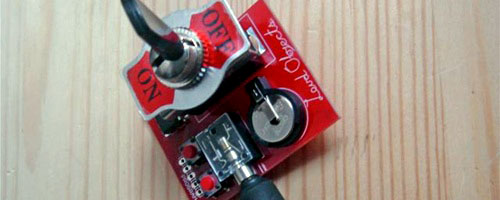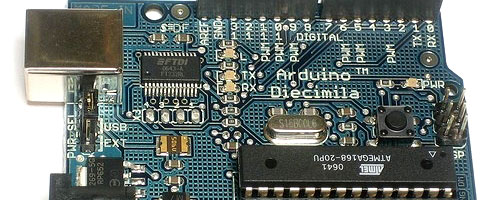Bit-music loving Arduino users – I ported (and modded a bit) one of the Noise Toy programs over to the lovely and talented Arduino platform. Just add a couple of momentary switches to pins 8 and 9 and connect stereo audio to 3 and 4 – you’re done. Loud Objects’ code proved quite fun to tinker/learn with – if you’re curious, try experimenting with different tempo values.Loud Objects Noise Toy kitArduino Diecimila

`/* Stereo BeatGen v1`

``` - originally written by Loud Objects for the ATTiny85/NOISE TOY projects loudobjects.com - adapted/messed with for Arduino by Collin Cunningham me [at] collinmel.com assumes pins 3 & 4 connected to left & right audio momentary switches between 8 & 9 and GND */ unsigned long NextRandom = 1; unsigned long Bass_Start = 300, Bass_End = 600; unsigned char Last_Val_Left = 1, Last_Val_Right = 1; unsigned long Bass_Left_Period = Bass_Start, Bass_Right_Period = Bass_Start; unsigned long Bass_Left_Sample = 1, Bass_Right_Sample = 1; unsigned char Bass_Left_On = 1, Bass_Right_On = 1; unsigned char Snare_Left_On = 1, Snare_Right_On = 1; unsigned char Snare_Left_Sample = 1, Snare_Right_Sample = 1; unsigned long Tempo_Period = 1200, Tempo_Outer = 1, Tempo_Sample = 1; unsigned char Trax_Left[] = { 1, 2, 3, 0, 3, 2, 0, 1}; unsigned char Trax_Right[] = { 2, 1, 0, 3, 1, 3, 0, 2}; unsigned char Track_Index = -1; unsigned long New_Rand = randomGen(); unsigned long NextRandom2 = 1; unsigned long Snare_Length = 500; void setup() { // SET PINS 3 AND 4 AS OUTPUTS pinMode(3, OUTPUT); pinMode(4, OUTPUT); pinMode(2, INPUT); // SET PULL-UP RESISTORS ON PINS 9 AND 8 pinMode(9, INPUT); pinMode(8, INPUT); digitalWrite(9, HIGH); digitalWrite(8, HIGH); digitalWrite(5, LOW); } void loop(){ NextRandom2 = NextRandom2 + 9; New_Rand = NextRandom2; // UPDATE SAMPLES if (Bass_Left_On) { if (!(--Bass_Left_Sample)) { if ((Bass_Left_Period += 11) > Bass_End) { Bass_Left_On = 0; } Bass_Left_Sample = Bass_Left_Period; Last_Val_Left = 1 - Last_Val_Left; } } if (Bass_Right_On) { if (!(--Bass_Right_Sample)) { if ((Bass_Right_Period += 13) > Bass_End) { Bass_Right_On = 0; } Bass_Right_Sample = Bass_Right_Period; Last_Val_Right = 1 - Last_Val_Right; } } if (Snare_Left_On) { if (!(--Snare_Left_Sample)) { Snare_Left_Sample = New_Rand / 200;// % 10; Last_Val_Left = 1 - Last_Val_Left; } if (!(--Snare_Length)) { Snare_Left_On = 0; } } if (Snare_Right_On) { if (!(--Snare_Right_Sample)) { Snare_Right_Sample = New_Rand / 200;// % 10; Last_Val_Right = 1 - Last_Val_Right; } if (!(--Snare_Length)) { Snare_Right_On = 0; } } // CHECK FOR NEXT BEAT if (!(--Tempo_Outer)) { Tempo_Outer = 10; if (!(--Tempo_Sample)) { if (++Track_Index == 8) Track_Index = 0; Tempo_Sample = Tempo_Period; Bass_Left_On = (Trax_Left[Track_Index] & 1); Bass_Right_On = (Trax_Right[Track_Index] & 1); Snare_Left_On = (Trax_Left[Track_Index] & 2); Snare_Right_On = (Trax_Right[Track_Index] & 2); Snare_Length = 5000; Bass_Left_Period = Bass_Start; Bass_Left_Sample = 1; Bass_Right_Period = Bass_Start; Bass_Right_Sample = 1; } } ```

``` // OUTPUT if (Last_Val_Left) PORTD |= (1 << 3); else PORTD &= ~((1 << 3)); if (Last_Val_Right) PORTD |= (1 << 4); else PORTD &= ~((1 << 4)); // BUTTON INPUT CHECKS //pin 8 for tempo if ((digitalRead(8)) == LOW) { randomSeed(analogRead(0)); Tempo_Period = (random(1627) / 15 * 25); // experiment with diff values for better range } //pin 9 for beat if (digitalRead(9) == LOW) { unsigned char i; for (i = 0; i < 8; i++) { Trax_Left[i] = randomGen(); Trax_Right[i] = randomGen(); } } } unsigned long randomGen(void) //pseudo random from original sketch { NextRandom = NextRandom * 3865 + 131; return ((NextRandom >> 16) & 32767); } ```

## 4 thoughts on “arduiNoise(beats);”

1.VanessaC says:

Hey, seems like a great project, very straightforward which is nice, its not too complicated to get started with! I found a great project here that’s similar, called the Arduino Synth: http://halfmachine.dk/posts/171

They say “We would like to make it possible to make installations which emits sounds and music without the need to have a computer connected. We needed to create a stand alone sound creation device which could be controlled by real time user input.” … “potentiometers are used to control the individual properties of each wave generator. In this way, sound can be controlled manually while playing music.”

Thanks for the post, nice work!

Vanessa

2.Collin Cunningham says:

@VanessaC – Nice find! Glad you enjoyed the post.